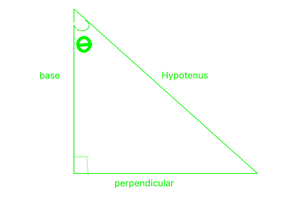# Find the exact value of tan 3π/4.

• Last Updated : 28 Sep, 2021

It is basically a study of the properties of the triangle and trigonometric function and their application in various cases. It helps in finding the angles and missing sides of a triangle with the help of trigonometric ratios. Commonly used angles are  0°, 30°, 45°, 60°, and 90°. With the help of only these angles, find the value of all other trigonometric angles.Hey! Looking for some great resources suitable for young ones? You've come to the right place. Check out our self-paced courses designed for students of grades I-XII

Start with topics like Python, HTML, ML, and learn to make some games and apps all with the help of our expertly designed content! So students worry no more, because GeeksforGeeks School is now here!

In this triangle, Given an acute angle θ,

• The sine of θ is written as sinθ and defined as the ratio sinθ = perpendicular/hypotenuse
• The cosine of θ is written as cosθ and defined as the ratio cosθ = base/hypotenuse
• The tangent of θ is written as tanθ and defined as the ratio tanθ = perpendicular/base = sinθ/cosθ

Note The reciprocals of sine, cosine, and tangents also have names: they are cosecant, secant, and cotangent.

• The cosecant of θ is written as cosecθ and defined as cosecθ = 1/sinθ
• The secant of θ is written as secθ and defined as secθ = 1/cosθ
• The cotangent of θ is written as cotθ and defined as cotθ = 1/tanθ

There are three Pythagorean Identities

1. sin2 θ + cos2 θ = 1
2. tan2 θ + 1 = sec2 θ
3. cot2 θ + 1 = cosec2 θ

Lets look at the complementary angles in trigonometric ratios,

• sin(90 + θ) = cosθ
• cos(90 + θ) = -sinθ
• tan(90 + θ) = -cotθ
• cot(90 + θ) = -tanθ
• sec(90 + θ) = -cosecθ
• cosec(90 + θ) = secθ

### Trigonometric Ratio Table

The trigonometric angles have a fixed value. Some of the important angles are used in mathematics. These fixed values are used in calculations. Let’s take a look at the table given below,

### Find the exact value of tan 3π/4.

Solution:

We have to find the value of tan3π/4

tan (3π/4) = tan(π/2 + π/4) = -cot(π/4) [as tan(90 + θ) = -cotθ here θ = π/4]

cot(π/4) = 1

So, tan(3π/4)=-1

Alternate Way

tan(180 – θ) = -tanθ

So, tan(3pi/4) = -tan(pi/4) = -1

### Similar Questions

Question 1: Find the value of tan(5π/6)

Solution:

⇒ tan(5π/6) = tan(π/2 + π/3) = -cot(π/3) [as tan(90 + θ) = -cotθ here θ = π/3]

so cot(π/3) = 1/√3

So, tan(5π/6) = -1/√3

Question 2: Find the value of tan(5π/4)

Solution:

⇒ tan(180 + θ) = tanθ [As tanθ is positive in the third quadrant]

S0, tan(5π/4) = tan(π + π/4) which is equal to tan(π/4)

tan(π/4) = 1

Hence, tan(5π/4) = 1

Question 3: What is the value of cot(5π/6)?

Solution:

⇒ We know that, cotθ = 1/tanθ

tan(5π/6) = -1/√3 [We have deduced earlier]

So, cot(5π/6) = -√3

Alternative way

⇒ cot(5π/6) = cot(π/2 + π/3) = -tan(π/3) [as cot(90 + θ) = -tanθ here θ = π/3]

So tan(π/3) = √3

Hence, cot(5π/6) = -√3

My Personal Notes arrow_drop_up